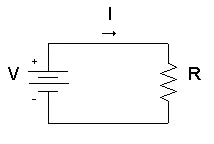# Power Calculator

Power consumption calculator: calculates electric power / voltage / current / resistance.

## DC power calculator

Enter 2 values to get the other values and press the Calculate button:

 Resistance (R): ohms (Ω) kilo-ohms (kΩ) mega-ohms (MΩ) Current (I): micro-amps (μA) milli-amps (mA) amps (A) kilo-amps (kA) mega-amps (MA) Voltage (V): micro-volts (μV) milli-volts (mV) volts (V) kilo-volts (kV) mega-volts (MV) Power (P): micro-watts (μW) milli-watts (mW) watts (W) kilo-watts (kW) mega-watts (MW)

#### DC power calculationVoltage (V) calculation from current (I) and resistance (R):

V(V) = I(A) × R(Ω)

Complex power (S) calculation from voltage (V) and current (I):

P(W) = V(V) × I(A) = V 2(V) / R(Ω) = I 2(A) × R(Ω)

## AC power calculator

Enter 2 magnitudes + 2 phase angles to get the other values and press the Calculate button:

 Impedance (Z): Ω kΩ MΩ ∠ ° = Current (I): μA mA A kA MA ∠ ° Voltage (V): V kV MV ∠ ° Power S: VA kVA MVA ∠ ° =

#### AC power calculation

So The voltage V in volts (V) is eqaul to the current I in amps (A) times the impedance Z in ohms (Ω).

V(V) = I(A) × Z(Ω) = (|I|×|Z|) ∠ (θI + θZ)

So The complex power S in volt-amps (VA) is equal to the voltage V in volts (V) times the current I in amps (A).

S(VA) = V(V) × I(A) = (|V|×|I|) ∠ (θV - θI)

So The real power P in watts (W) is equal to the voltage V in volts (V) times current I in amps (A) times the power factor (cos φ).

P(W) = V(V) × I(A) × cos φ

So The reactive power Q in volt-amps reactive (VAR) is equal to the voltage V in volts (V) times the current I in amps (A) time the sine of the complex power phase angle (φ).

Q(VAR) = V(V) × I(A) × sin φ

So The power factor (FP) is equal to the absolute value of the cosine of the complex power phase angle (φ).

PF = |cos φ|

## Energy & power calculator

Enter 2 values to get the other values and press the Calculatebutton:

 Energy: J Time period s Average power: W

#### Energy & power calculation

So The average power P in watts (W) is equal to the energy consumed E in joules (J) divided by time period Δ t in seconds (s).

P(W) = E(J) / Δ t(s)

Electric power ►

## Features of Power conversion

Our Power conversion allows the users to Calculate Power. Some of the prominent features of this utility are explained below.

### No Registration

You don’t need to go through any registration process to use the Power converter. Using this utility, you can convert Power as many times as you want for free.

#### Fast conversion

This Power Calculator offers users the fastest conversion. Once the user enters the  Power values ​​in the input field and clicks the Convert button, the utility will start the conversion process and return the results immediately.

#### Saves Time and Effort

The manual procedure of Calculator Power isn’t an easy task. You must spend a lot of time and effort to complete this task. The Power Calculator allows you to complete the same task immediately. You will not be asked to follow manual procedures, as its automated algorithms will do the work for you.

##### Accuracy

Despite investing time and effort in manual Calculation, you might not be able to get your hands on accurate results. Not everyone is good at solving math problems, even if you think you're a pro, there's still a good chance you'll get in accurate results. This situation can be smartly handled with the help of a Power Calculator. You will be provided with 100% accurate results by this online tool.

##### Compatibility

The online Power converter perfectly works on all operating systems. Whether you have a Mac, iOS, Android, Windows, or Linux device, you can easily use this online Tool without facing any hassle.

###### 100% Free

You don't need to go through any registration process to use this Power Calculator. You can use this utility for free and do unlimited Power conversions without any limitations.